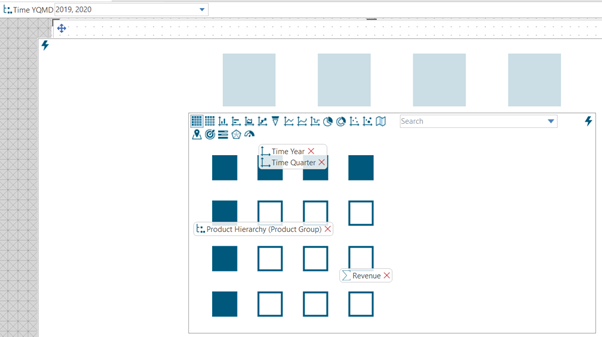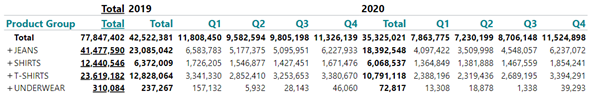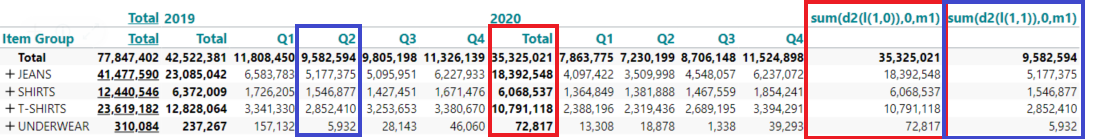# Addressing levels in hierarchies #2

In the article (Addressing levels in hierarchies 1), I explained a few basic facts about addressing levels in a predefined hierarchy in TARGIT.

In this article I will take it a bit further – here’s the definition of a table with multiple attributes on the horizontal axis, none of them being a hierarchy – but together they make a custom hierarchy:In terms of levels things are different now compared to a “real” hierarchy

• Year – level 1 (now expressed(l(1,0))
• Quarter – level 1 (now expressed(l(1,1))
(but you don’t have to use any level parameters if you mean “the lowest level” – that’s default anyway)

The resulting table will look something like this:So let’s make the same calculation as in the previous article (Addressing levels in hierarchies 1) and make a Year Growth calculation.

However the formula we used before (Sum(d2(l1),0,m1)-sum(d1(l1),0,m1)) will NOT work now. That’s because it’s a custom hierarchy.

The syntax will now be this: sum(d2(l(1,0)), 0, m1) - sum(d1(l(1,0)), 0, m1).

So what you can learn from this article and the previous one is that there’s a big difference between levels in predefined hierarchies and custom hierarchies.

More to come on addressing levels...

1 out of 1 found this helpful

• Hi Niels,

It would be very helpful if you explain what the parts of the syntax you add, as everyone will need to adapt it to their own hierarchy.

The syntax for the "real" hierarchy makes sense, as it says dimension d2 sum for level 1.

But what does the other one do? d2(l(1,0)) ? its essential for knowing how to exchange the numbers.

I want to use it for summing product groups, if you extend it so see items in the groups in this example :-)

• Hi Lara

If you have 2 attributes on the same axis, they form a custom hierarchy.

sum(d2(l(1,0)),0,m1) in my example means something like:

The second column (d2) - level 1 on the first attribute (Year) and level 0 on the second attribute (Quarter).

Level 1 is the default level - so column d2 level 1 on the year just means the second year -

Level 0 is a little harder to get your head around - it's often called the "all" level - but what is important here, is that it means no particular quarter (or you could say - "just ignore quarters").

The table below might be helpfull in understanding these subtle differences:D2 level 1,0 means the YEAR 2020 (the second year)

D2 level 1,1 means the QUARTER Q2 in 2019 (the second quarter).

In my example I'm only referring to levels on columns, while the row reference just says current row (0). - so if you expand the product groups it won't make a different in the level references.

Hope it makes sense :-)

br/Niels